## NCERT Solutions for Class 12 Micro Economics Chapter – 3 Demand

### NCERT TEXTBOOK QUESTIONS SOLVED

Question 1. Suppose there are two consumers in the market for a good and their demand functions are as follows: $${ D }_{ 1 }( p) =20-P$$ for any price than less 20, and $${ D }_{ 1 }(p)=0$$ at any price greater than or equal to 20. $${ D }_{ 2 }(p)=30-2P$$ for any price less than 15 and $${ D }_{ 2 }(p)=0$$ at any price greater than or equal to 15. Find out the market demand function.
[3-4 Marks]
Answer: It can be seen from the given demand functions that Consumer 1 do not want to demand the goods for any price greater than or equal Rs.20 and consumer 2 do not want to demand the goods for any price greater than Rs 15.
Hence, the market demand function will be,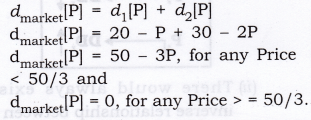Question 2. Suppose there are 20 consumers for a good and they have identical demand function?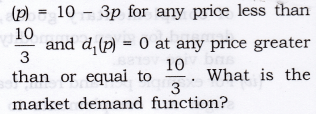Question 3.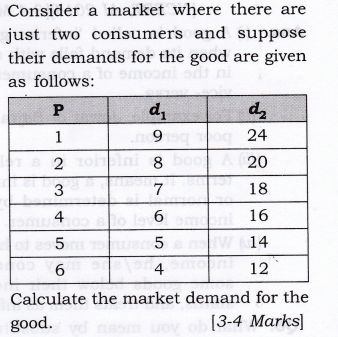Question 4. What do you mean by a normal good?(or)
when good is called ‘normal goods’?
Answer: for normal good, with a raise in income,the demand of the commodity also rises and vice versa. Shortely direct relationship exists the income of a coustomer and demand of normal good.For example, a new car, new clothings.

Question 5. What do you mean by an ‘inferior good’? Give some examples. [CBSE 2006]
Or
Give the meaning of inferior good and explain the same with the help of an example.[NCERT, AI 2014] [3-4 Marks]

1. A good is called ‘inferior goods’ when its demand falls with a rise in the income of a consumer and vice- versa.
2. For example, Jowar or Bajra for a poor person.
3. A good is inferior in a relative terms. It means, a good is inferior or normal is determined by the income level of a consumer.
4. When a consumer moves to higher income, he/she may consider some goods below their income status, and treats them as inferior.

Question 6. What do you mean by substitutes? Give examples of two goods which are substitutes of each other. [3-4 Marks]

1. Substitute goods are those goods which can be used in place of another goods and give the same satisfaction to a consumer.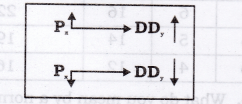2.  There would always exist a direct relationship between the price of substitute goods and demand for given commodity.
3.  It means with an increase in price of substitute goods, the demand for given commodity also rises and vice-versa.
4. For example, Pepsi and Coke, tea and coffee are substitute to each other.

Question 7. What do you mean by complements?
Give examples of two goods which are complements of each other. [3-4 Marks]

1.  Complementary goods are those which are useless in the absence of other goods and which are demanded jointly.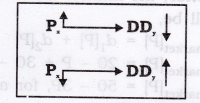2. There would always exist an inverse relationship between price of complementary goods and demand for given commodity.
3.  It means, with a rise in price of complementary goods, the demand for given commodity falls and vice-versa.
4.  For example pen and refill, tea and sugar are complements to each other.

### MORE QUESTIONS SOLVED

I. Very Short Answer Type Questions  (1 Mark)
Question 1. What is meant by demand? [CBSE 2005C, AI 07]
Answer: Demand is a quantity of a commodity that a consumer wishes to purchase at a given level of price and during a specified period of time.

Question 2. Define market demand. [CBSE 2008, 12, 13]
Answer: Market demand refers to the quantity of a commodity that all the consumers are willing and able to buy, at a particular price during a given period of time.

Question 3. Due to rise in price of commodity x the demand of commodity y falls. What type of commodity are they?

Question 4. Due to rise in price of the commodity x, the demand of commodity y also rises. What type of commodity they are?

Question 5. How will an increase in the price of petrol affect the demand curve of a car?
Answer: The demand curve of a car will shift to the left.

Question 6. A fall in the income of the consumer leads to a rise in the demand for a good. What is good X called?

Question 7. What is meant by the law of demand?
Answer: It states that price of the commodity and quantity demanded are inversely related to each other when other factors remain constant (ceteris Paribus).

Question 8. When the demand for a good rises due to a fall in its own price, what is the change in demand called?

Question 9. Define ‘change in demand’.[CBSE Sample Paper 2008]
Answer: If demand changes due to the change in factors other than price, it is known as change in demand.

Question 10. What causes an upward movement along a demand curve of a commodity?
[CBSE Sample Paper 2010]
Answer: Rise in price of goods and fall in quantity demanded i.e., Contraction in demand.

Question 11. Give one reason for a shift in demand curve. [AT 2012]
Answer: Change in price of substitute goods.

Question 12. What determines the quantity of a good that the buyers demand for?
Answer: The quantity of a good that the buyers demand for is determined by the price of the goods, income, the prices of related goods, tastes, expectations, and the number of buyers.

Question 13. Why market demand curve is flatter?
Answer:  Market demand curve is flatter than the individual demand curves because as price falls, proportionate rise in market demand is more than proportionate rise in individual

Question 14. Ceteris Paribus, if the government provides subsidies on electricity bills, what would be the likely change in the market demand of desert coolers?[CBSE Sample Paper 2016]
Answer: Market demand for desert coolers will Increase.

II. Multiple Choice Questions (1 Mark)
Question 1. Demand for a commodity refers to:
(a) desire for the commodity.
(b) need for the commodity.
(c) quantity demanded of that commodity.
(d) quantity of the commodity demanded at a certain price during any particular period of time.

Question 2. Contraction of demand is the result of:
(a) decrease in the number of consumers.
(b) increase in the price of goods concerned.
(c) increase in the prices of other goods.
(d) decrease in the income of purchasers.

Question 3. All but one of the following are assumed to remain the same while drawing an individual’s demand curve for a commodity. Which one is it?
(a) The preference of the individual.
(b) His monetary income.
(c) Price.
(d) Price of related goods.

Question 4. Which of the following pairs of goods is an example of substitutes?
(a) Tea and sugar.
(b) Tea and coffee.
(c) Pen and ink.
(d) Shirt and trousers.

Question 5. The Law of Demand, assuming other things to remain constant, establishes the relationship between:
(a) income of the consumer and the quantity of goods demanded by him.
(b) price of goods and the quantity demanded.
(c) price of goods and the demand for its substitute.
(d) quantity demanded of goods and the relative prices of its complementary goods.

Question 6. If regardless of changes in its price, the quantity demanded of a good remains unchanged, then the demand curve for the good will be:
(a) horizontal. (b) vertical.
(c) positively sloped.
(d) negatively sloped.

Question 7. Suppose the price of Pepsi increases, we will expect the demand curve of Coca-Cola to:
(a) shift towards left.
(b) shift towards right
(c) initially shift towards left and then to right.
(d) remains at the same level.

Question 8.  All of the following items are determinants of demand except:
(a) tastes and preferences.
(b) quantity supplied.
(c) income.
(d) price of related goods.

Question 9. A movement along the demand curve for soft drinks is best described as:
(a) an increase in demand.
(b) a decrease in demand.
(c) a change in quantity demanded.
(d) a change in demand.

Question 10. If the price of Pepsi decreases relative to the price of Coke and 7-UP, the demand for:
(a) Coke will decrease.
(b) 7-UP will decrease.
(c) Coke and 7-UP will increase.
(d) Coke and 7-UP will decrease.

Question 11. The price of tomatoes increases and people buy tomato puree. You infer that tomato puree and tomatoes are:
(a) normal goods (b) complements
(c) substitutes (d) inferior goods

Question 12. Potato chips and popcorn are substitutes. A rise in the price
of potato chips will the
demand for popcorn and the demand of potato chips will
(a) increase; increase
(b) increase; decrease
(c) decrease; decrease
(d) decrease; increase

Question 13. When total demand for a commodity whose price has fallen increases, it is due to:
(a) income effect
(b) substitution effect
(c) complementary effect
(d) price effect

Question 14. With a fall in the price of a commodity:
(a) consumer’s real income increases
(b) consumer’s real income decreases
(c) there is no change in the real income of the consumer
(d) None of these.

Question 15.  Goods that exhibit direct price- demand relationship are called:
(a) Geffen goods
(b) complementary goods
(c) substitute goods
(d) None of these.

Question 16.  In case of Giffen goods, the demand curve will be:
(a) horizontal.
(b) downward-sloping to the right.
(c) vertical.
(d) upward-sloping to the right.

Question 17. Law of Demand is a:
(a) quantitative statement
(b) qualitative statement
(c) Both (a) and (b)
(d) None of these.

Question 18. When income of the consumer falls the impact on price-demand curve of an inferior good is: [CBSE 2015]
(a) Shifts to the right.
(b) Shifts to the left.
(c) There is upward movement along the curve.
(d) There is downward movement along the curve.

Question 19. If due to fall in the price of good X, demand for good Y rises, the two goods are: [AI 2015]
(a) Substitutes (b) Complements
(c) Not related (d) Competitive

III. Short Answer Type Questions (3-4 Marks)
Question 1. Does a rise in price of other goods have the same effect on demand for a commodity?
Answer: No, rise in prices of other goods does not have the same effect on demand for a commodity.

1.  In case of rise in price of substitute goods, demand for the given commodity rises.
2. In case of rise in price of complementary goods, demand for the given commodity falls.

Question 2. Does a fall in income have the same effect on demand for the given commodity?
Answer: No, fall in income does not have the same effect on demand for the given commodity.

1. If the given commodity is a normal good, the fall in income will reduce the demand for the normal goods.
2. If the given commodity is an inferior good, the fall in income will raise the demand for the inferior goods.
3. If the given commodity is a necessity, the fall in income will not change the demand for the necessity of goods.

Question 3. What is the relation between good x and good y in each case, if with a fall in price of x demand for good y (1) rises and (2) falls? Give reason.[CBSE 2008}

1. Goods x and y are complementary goods as with fall in price of x, demand for good y rises.
2. Goods x and y are substitute goods as with the fall in price of x, demand for good y also falls.

Question 4. Giving reasons, state if the following statements are true or false:

1. An increase in the price of Coke would result in decrease in the demand for Pepsi.
2. An increase in the price of sugar would result in an increase in the demand for tea.
3. An increase in the income of a consumer would result in an increase in demand for all types of goods that are demanded by a consumer.

1. False: Coke and Pepsi are substitute goods. An increase in the price of Coke would induce consumers to substitute Coke by Pepsi. Demand for Pepsi will increase.
2.  False: Sugar and tea are complementary goods. An increase in prite of sugar would make tea costlier. Hence, the demand for tea would decrease.
3. False: With the increase in income, the consumer’s demand for normal goods will increase. Demand for inferior goods may, in fact, fall.

Question 5. Differentiate between Normal Goods and Inferior Goods. [AI 2012, CBSE 2013]Question 6. Differentiate between substitute goods and complementary goods.Question 7. Explain law of demand with the help of a demand schedule.[AI 2005, 09]

1. It states that price of the commodity and quantity demanded are inversely related to each other, when other factors remain constant.
It means, quantity demanded of the commodity rises due to fall in price of the commodity and vice-versa.
2. Ceteris Paribus means:
(a) Price of Related commodity remains constant.
(b) Income of a consumer remains constant.
(c) Taste and preferences of a household remains constant.3. The law of demand makes a qualitative statement only and not quantitative. It indicates the direction of change in the amount demanded and it does not indicate the magnitude of change.
4. Law of demand is one sided. It explains only the effect of change in price on the quantity demanded. It states nothing about the effect of change in quantity demanded on the price of the commodity.

Question 8. Under what conditions a consumer would like to demand more at a given level of price?
Or
State any three causes of a rightward shift of a demand curve of a commodity.[CBSE 2005, 06, 09, AI 2005] Or
State any three factors which lead to increase in demand.[CBSE 2004C, AI 10]1. Price of substitute goods rises.
2. Price of complementary goods falls.
3. Income of a consumer rises in case of normal goods.
4. Income of a consumer falls in case of inferior goods.
5. When the preferences are favourable.

Question 9. Under what conditions a consumer would like to demand less at a given level of price?
Or
State any three causes of a leftward shift of a demand curve of a commodity. [CBSE 2006]
Or
State any three factors which lead to decrease in demand. [AI 2004]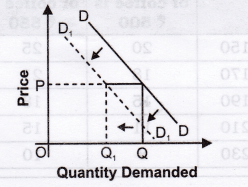1. Price of substitute goods falls.
2. Price of complementary goods rises.
3. Income of a consumer falls in case of normal goods.
4. Income of a consumer rises in case of inferior goods.
5. When a preference becomes unfavourable.

Question 10. Differentiate between increase in demand and expansion in demand (increase in quantity demanded). [CBSE 2003, 2010]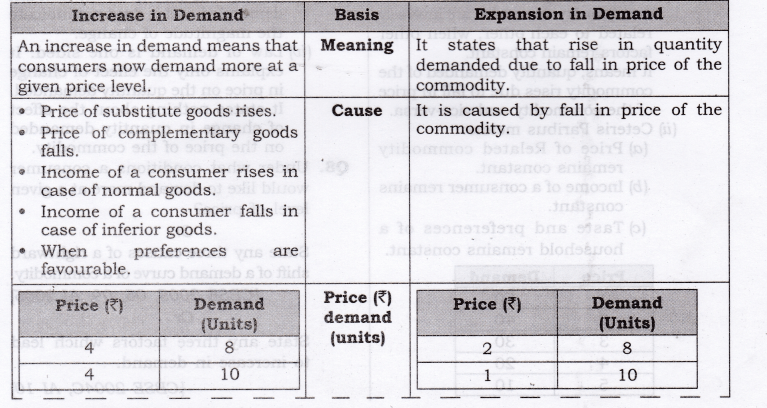Question 11. Differentiate between decrease in demand and contraction in demand (decrease in quantity demanded). [AI 2003]Question 12. Explain the inverse relationship between the price of a commodity and its demand. [AI 2010, CBSE Sample Paper 2010, CBSE 2000, AI 2000]
Or
Why does law of demand slope downward from left to right?
Or
Why do household buy more at a lower price than at a higher price?
Or
Explain the causes behind law of demand.
Answer: The inverse relationship between price of the commodity and quantity demanded for that commodity is because of the following reasons:

1. Income effect:
(a) Quantity demanded of a commodity changes due to change in purchasing power (real income), caused by change in price of a commodity is called Income Effect,
(b) Any change in the price of a commodity affects the purchasing power or real income of the consumers although his money income remains the same.
(c) When price of a commodity rise more has to be spent on purchase of the same quantity of that commodity. Thus, rise in price of commodity leads to fall in real income, which will thereby reduce quantity demanded is known as Income effect.
2. Substitution effect:
(a) It refers to substitution of one commodity in place of another commodity when it becomes relatively cheaper.
(b) A rise in price of the commodity let coke, also means that price of its substitute, let pepsi, has fallen in relation to that of coke, even though the price of pepsi remains unchanged. So, people will buy more of pepsi and less of coke when price of coke rises.
(c) In other words, consumers will substitute pepsi for coke. This is called Substitution effect.
Price effect = Income effect + Substitution effect
3. Law of Diminishing Marginal Utility:
(a) This law states that when a consumer consumes more and more units of a commodity, every additional unit of a commodity gives lesser and lesser satisfaction and marginal utility decreases.
(b) The consumer consumes a commodity till marginal utility (benefit) he gets equals to the price (cost) they pay, i.e., where benefit = cost.
(c) For example, a thirsty man gets the maximum satisfaction (utility) from the first glass of water. Lesser utility from the 2nd glass of water, still lesser from the 3rd glass of water and so on. Clearly, if a consumer wants to buy more units of the commodity, he would like to do so at a lower price. Since, the utility derived from additional unit is lower.
(a) When price of a commodity falls, two effects are quite possible:
• New consumers, that is, consumers that were not able to afford a commodity previously, starts demanding it at a lower price.
• Old consumers of the commodity starts demanding more of the same commodity by spending the same amount of money.
(b) As the result of old and new buyers push up the demand for a commodity when price falls.

IV. True Or False
Giving reasons, state whether the following statements are true or false.
Question 1. Law of demand states that price and demand are positively related to each other.
Answer: False: Law of demand states that price and demand are inversely related to each other.

Question 2. Law of demand happens due to application of law of diminishing marginal productivity.
Answer: False: It is due to application of law of diminishing marginal utility.

Question 3. Law of demand explains quantitative relationship between price and quantity demanded.
Answer: False: Law of demand explains qualitative relationship between price and demand.

Question 4. Change in quantity demanded means an increase and decrease in demand and Change in demand curve means expansion and contraction in demand.
Answer: False: Change in quantity demanded means expansion and contraction in demand and change in demand curve means increase and decrease in demand.

Question 5. With a fall in income, demand for normal goods will rise.
Answer: False: With a fall in income, demand for normal goods will decrease because of positive relationship between income and demand for normal goods.

Question 6. With the rise in price, quantity demanded for the goods falls and it is known as decrease in demand.
Answer: False: With a rise in price, quantity demanded falls and it is known as contraction in demand. It is not. an decrease in demand as it changes due to factors other than price.

Question 7. If price of substitute goods falls, demand for related goods will also fall?
Answer: True: With a fall in price of substitute goods, demand for its related goods will fall as there exists positive relationship between them.

Question 8. If income of the buyer decreases, demand for inferior goods decreases.
Answer: False: When income of the buyer decreases , demand for inferior goods increases. It is so because inverse relationship exists between them.

Question 9. If Indian Airlines reduce fares from Delhi to Mumbai, demand curve for air travel shifts rightward.
Answer: False: It is due to price change. It is expansion and not increase in demand.
Note: As per CBSE guidelines, no marks will be given if reason to the answer is not explained.

V. Long Answer Type Questions (6 Marks)
Question 1. Explain with the help of diagrams, the effect of the following changes on the demand of a commodity:
(i) A rise in price of Substitute good. [ CBSE 2005, 08C; AI08]
(ii) A rise in price of Complementary good. [AI 2004; CBSE 06C]

1. A rise in price of Substitute good
(a) Substitute goods are the goods that can be used in place of another goods and give the same satisfaction to a consumer.(b) There would always exist a direct relationship between the price of substitute goods and demand for given commodity.
(c) Due to rise in price of substitute (Say Coffee from Rs. 500 to Rs. 550), the demand of tea shift rightward from DD to D1D1 as shown in given figure:
2. A rise in price of Complementary good(a) Complementary goods are those which are useless in the absence of another good and which are demanded jointly.
(b) There would always exist an inverse relationship between price of complementary goods and demand for given commodity.
(c) Due to rise in price of Complementary good (Say Tea from Rs 500 to Rs 550), the demand of Sugar shift leftward from DD to D1D1 as shown in given figure.

Question 2. Explain with the help of diagrams, the effect of the following changes on the demand of a commodity:
(i) A fall in price of Substitute good. [CBSE 2004, CBSE 07C]
(ii) A fall in price of Complementary good.[CBSE 2006C, 2005, 08C; AI 08]

1. A fall in price of Substitute good: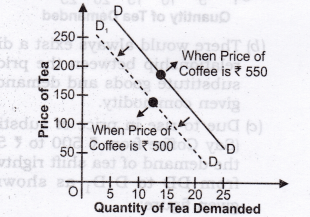(a) Substitute goods are the goods that can be used in place of another goods and give the same satisfaction to a consumer.
(b) There would always exist a direct relationship between the price of substitute goods and demand for given commodity.
(c) Due to fall in price of substitute (Say Coffee from Rs 550 to Rs 500), the demand of tea shift leftward from DD to D1D1 as shown in adjacent figure:
2. A fall in price of Complementary good(a) Complementary goods are those which are useless in the absence of another good and which are demanded jointly.
(b) There would always exist an inverse relationship between price of complementary goods and demand for given commodity.
(c) Due to fall in price of complementary good (Say Tea from Rs 550 to Rs 500), the demand of sugar shift rightward from DD to D1D1 as shown in given figure.

Question 3. Explain the effect of the following on demand for a good:
(i) Rise in Income for Normal Goods. [AI 2004, 08, CBSE 06C, 07, 09C, IOC]
(ii) Rise in Income for Inferior Goods. [AI 2004, 08, CBSE 06C, 07, 09C, 10]

1. Rise in Income for Normal Goods:(a) For normal commodity, with a rise in income, the demand of the commodity also rises and vice- versa.
(b) Shortly, direct relationship exists between income of a consumer and demand of normal commodity.
(c) Due to rise in income of a consumer (Say from Rs 5000 to Rs 6000), the demand of normal goods shifts rightward from DD to D1D1 as shown in above figure.
2. Rise in Income for Inferior Goods: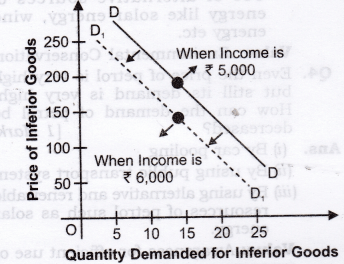(a) For inferior goods, with a rise in income, the demand of the commodity falls and vice-versa.
(b) Shortly, inverse relationship exists between income of a consumer and demand of inferior goods.
(c) Due to rise in income of a consumer (say from Rs. 5000 to Rs. 6000), the demand of inferior goods shifts leftward from DD to D, D j as shown in adjacent figure:

Question 4. Explain the effect of the following on demand for a good:
(i) Fall in Income for Normal Goods. [CBSE 2004, 09C, AI 06C]
(ii) Fall in Income for Inferior Goods. [CBSE 2004, 09C, AI 06C]

1.  Fall in Income for Normal Goods: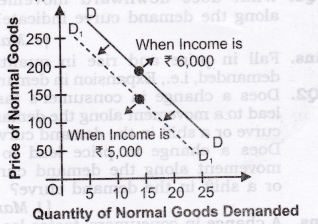(a) For normal commodity, with a fall in income, the demand of the commodity also falls and vice- versa.
(b) Shortly, direct relationship exists between income of a consumer and demand of normal commodity.
(c) Due to fall in income of a consumer (Say from Rs 6000 to Rs 5000), the demand of normal goods shifts leftward from DD to D1D1 as shown in figure above.
2. Fall in Income for Inferior Goods:(a) For inferior goods, with a fall in income, the demand of the commodity rises and vice-versa.
(b) Shortly, inverse relationship exists between income of a consumer and demand of inferior goods.
(c) Due to fall in income of a consumer (Say from Rs.6000 to Rs.5000), the demand of inferior goods shifts rightward from DD to D1D1 as shown in figure.

VI. Higher Order Thinking Skills
Question 1. What does downward movement along the demand curve indicate? [1 Mark]
Answer: Fall in price and rise in quantity demanded, i.e., Expansion in demand.

Question 2. Does a change in consumer’s taste lead to a movement along the demand curve or a shift in the demand curve? Does a change in price lead to a movement along the demand curve or a shift in the demand curve? [1 Mark]
Answer: A change in consumers’ tastes leads to a shift of the demand curve. A change in price leads to a movement along the demand curve.

Question 3. Law of Demand is a qualitative statement. Comment. [3-4 Marks]

1. Law of demand is only an qualitative, and not a quantitative statement.
2. It indicates only the direction in which the quantity demanded will change with a change in price. It says nothing about the magnitude of such a change.
3. For example, price of coke rises from Rs. 8 to Rs. 10 per bottle, then as per law of demand, we can say that the demand for coke will fall. But the law does not give the actual amount by which the demand for coke will decline.

VII. Value Based Questions
Question 1. According to law of demand by increasing the price of a good its demand decreases but in case of petrol, its demand increases with the increase in price why? Explain. [1 Mark]
Answer: Nowadays petrol has become a necessary good and its supply is limited.
Value: Critical thinking

Question 2. What policy initiatives can the government undertake to increase the demand of milk in the country? Mention any one. [1 Mark] [CBSE Sample Paper 2014]
Answer: Give subsidies to reduce price. (Any 1) or
Undertake health campaigns to promote the positive effects of milk consumption.
Value: Analytic

Question 3. Demand for electricity has “increased”. However supply cannot be increased due to lack of resources. Explain how, in any two ways, demand for electricity can be “decreased”. [3 Marks]
Answer: Electricity is a public utility service therefore its increased demand should not be reduced. However, supply cannot be immediately increased due to lack of resources. Therefore, in the given situation, ways should be found to meet the increased demand of the electricity from available supply of the electricity.
Demand for electrical appliances:

• Use of energy saving electrical appliances.
• Use of alternative sources of energy like solar energy, wind energy etc.

Value: Environmental Conservation

Question 4. Even the price of petrol is very high but still its demand is very high. How can the demand of petrol be decreased? [ 1 Mark]

1.  By car pooling
2. By using public transport system
3. By using alternative and renewable resources of petrol such as solar energy.

Value: Awareness for efficient use of resources

VIII. Application Based Questions
Question 1. A new steel plant comes up in Jharkhand. Many people who were previously unemployed in the area are now employed. How will this affect demand curve for TV? [ 1 Mark]
Answer:  The demand curve for TV will shift towards right. It happens because of increase in the income of the people due to employment in new steel plant.

Question 2. In order to encourage tourism in Goa, Indian Airlines reduces the air fare to Goa. How will it affect market demand curve for air travel to Goa?[1 Mark]
Answer: There will be a downward movement along the same market demand curve (expansion in demand) for air travel to Goa. It happens because of decrease in the air fare.

Question 3. There are train and bus services between New Delhi and Jaipur. Suppose that the train fare between the two cities comes down. How will this affect demand curve for bus travel between the two cities?[1 Mark]
Answer: Demand curve for bus travel will shift towards left. It happens because price of substitute (train fare) has decreased and it will make the bus travel relatively costly.

Question 4.  A good is an ‘inferior’ good for one and at the same time a ‘normal’ good for another consumer. Do you agree? Explain. [3-4 Marks] [CBSE Sample Paper 2013, 2014]

1.  Yes, the same goods can be inferior for one and normal for another one.
2.  Whether a good is inferior or normal is determined by the income level of a consumer.
3. A good is a normal good for the consumer having lower income, may become an inferior good for a consumer having higher income.
4. When a consumer moves to higher income, he/she may consider some goods below their income status, and treats them as inferior.

Question 5. Normally a household will not buy a second-hand scooter even if the price of scooter falls. How we get a downward sloping market demand curve for scooters? [3-4 Marks]